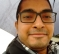## LTE WiFi FFT ProblemStarted by 5 years ago4 replieslatest reply 5 years ago162 views

Hi,

Suppose I have time domain LTE signal L and time domain WiFi signal W.

L is generated by taking 2048 point IFFT and W is generated by taking 64 point FFT.

Power of L is higher than W in such a way that after adding them, i.e. , L+W and taking 2048 point FFT, the LTE can still be detected and decoded.

Now here is the situation. I received time domain L+W, took 2048 point FFT on L+W denoted by F(L)+F(W).

Now we decode LTE and after that perform some signal processing on F(L)+F(W) in such way that F(L) is perfectly nulled  out from F(L)+F(W). In other words interference nulling of LTE from composite signal. After such nulling the residue is F(W). Now if I take 2048 point IFFT of F(W) to get a time domain, i.e., W and then take 64 point FFT of W, i.e., F(W), will I be able to recover WiFi (assuming that nulling of LTE has worked perfectly) from F(W).

Saying simply if F64/F2048 and IF64/IF2048 are 64/2048 point FFT and IFFT operators

then, will F64( IF2048 ( F2048 ( IF64 (A))))  be equal to A ?

Regards

Sumit

[ - ]Well I tried in Matlab:

a = randn(1,64);

b = fft(ifft(fft(ifft(a,64),2048),2048),64);

plot(a); hold;

plot(real(b),'r--');

result is that a = real(b) apart from rounding issues

[ - ]Indeed! I also replicated and this happens without zero-padding.

I was in the impression that taking 2048 point FFT of the LTE+WiFi will introduce some ICI in the WiFi sub carriers which WiFi will not able to recover later.

[ - ]I think there must be a stage of zero padding:

64 points ifft to 2048 fft requires zero adding in center

2048 fft to 2048 ifft, no zero padding

2048 ifft to 64 fft, no zero padding, decimation needed

[ - ]